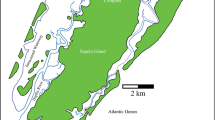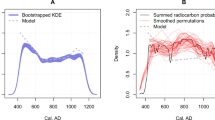# Regression analysis with partially labelled regressors: carbon dating of the Shroud of Turin

## Abstract

The twelve results from the 1988 radio carbon dating of the Shroud of Turin show surprising heterogeneity. We try to explain this lack of homogeneity by regression on spatial coordinates. However, although the locations of the samples sent to the three laboratories involved are known, the locations of the 12 subsamples within these samples are not. We consider all 387,072 plausible spatial allocations and analyse the resulting distributions of statistics. Plots of robust regression residuals from the forward search indicate that some sets of allocations are implausible. We establish the existence of a trend in the results and suggest how better experimental design would have enabled stronger conclusions to have been drawn from this multi-centre experiment.

This is a preview of subscription content, log in via an institution to check access.

Price excludes VAT (USA)
Tax calculation will be finalised during checkout.

### Similar content being viewed by others### The Case for Radiocarbon Dating and Bayesian Analysis in Historical Archaeology

Victor D. Thompson, Richard W. Jefferies & Christopher R. Moore### On Applications of Space–Time Modelling with Open-Source 14C Age Calibration

T. Rowan McLaughlinE. R. Crema

## References

• Abraham, B., Box, G.E.P.: Linear models and spurious observations. Appl. Stat. 27, 131–138 (1978)

• Atkinson, A.C., Riani, M.: Robust Diagnostic Regression Analysis. Springer, New York (2000)

• Atkinson, A.C., Riani, M., Cerioli, A.: The forward search: theory and data analysis (with discussion). J. Korean Stat. Soc. 39, 117–134 (2010). doi:10.1016/j.jkss.2010.02.007

• Bailey, R.A., Nelson, P.R.: Hadamard randomization: a valid restriction of random permuted blocks. Biom. J. 45, 554–560 (2003)

• Ballabio, G.: (2006). New statistical analysis of the radiocarbon dating of the Shroud of Turin. Unpublished manuscript. See http://www.shroud.com/pdfs/doclist.pdf

• Box, G.E.P.: Non-normality and tests on variances. Biometrika 40, 318–335 (1953)

• Box, G.E.P., Hunter, W.G., Hunter, J.S.: Statistics for Experimenters. Wiley, New York (1978)

• Buck, C.E., Blackwell, P.G.: Formal statistical models for estimating radiocarbon calibration curves. Radiocarbon 46, 1093–1102 (2004)

• Christen, J.A.: Summarizing a set of radiocarbon determinations: a robust approach. Appl. Stat. 43, 489–503 (1994)

• Christen, J.A., Sergio Perez, E.: A new robust statistical model for radiocarbon data. Radiocarbon 51, 1047–1059 (2009)

• Damon, P.E., Donahue, D.J., Gore, B.H., et al.: Radio carbon dating of the Shroud of Turin. Nature 337, 611–615 (1989)

• Fanti, G., Botella, J.A., Di Lazzaro, P., Heimburger, T., Schneider, R., Svensson, N.: Microscopic and macroscopic characteristics of the Shroud of Turin image superficiality. J. Imaging Sci. Technol. 54, 040201 (2010)

• Freer-Waters, R.A., Jull, A.J.T.: Investigating a dated piece of the Shroud of Turin. Radiocarbon 52, 1521–1527 (2010)

• Müller, W.G.: Collecting Spatial Data, 3rd edn. Springer, Berlin (2007)

• Ramsey, C.B.: Dealing with outliers and offsets in radiocarbon data. Radiocarbon 51, 1023–1045 (2009)

• Reimer, P.J., Baillie, M.G.L., Bard, E., et al.: INTCAL04 terrestrial radiocarbon age calibration. Radiocarbon 46, 1029–1058 (2004)

• Rousseeuw, P.J.: Least median of squares regression. J. Am. Stat. Assoc. 79, 871–880 (1984)

• Sacks, J., Welch, W.J., Mitchell, T.J., Wynn, H.P.: Design and analysis of computer experiments. Stat. Sci. 4, 409–435 (1989)

• Walsh, B.: The 1988 Shroud of Turin radiocarbon tests reconsidered. In: Walsh, B. (ed.) Proceedings of the 1999 Shroud of Turin International Research Conference, Richmond, Virginia, USA, pp. 326–342. Magisterium Press, Glen Allen (1999)

## Author information

Authors

### Corresponding author

Correspondence to Anthony C. Atkinson.

## Appendix: Weighted and unweighted analyses

### Appendix: Weighted and unweighted analyses

The data suggest three possibilities for the weights v ij in (1):

1. Unweighted Analysis. Standard analysis of variance: all v ij =1.

2. Original weights. We weight all observations by 1/v ij , where the v ij are given in Table 1. That is, we perform an analysis of variance using responses z ij =y ij /v ij . If these v ij are correct, in (1) σ=1 and the total within groups sum of squares in the analysis of variance is distributed as χ 2 on 9 degrees of freedom, with the expected mean squared error being equal to one.

3. Modified weights. The v ij for the TS from Arizona in Table 1 are very roughly 2/3 of those for the other sites. The text above Table 1 of Damon et al. (1989) indicates that the weights for Arizona include only two of the three additive sources of random error in the observations. Table 2 of their paper gives standard deviations for the mean observation at each site calculated to include all three sources. In terms of the v ij the standard deviations of the means are

$$\mbox {s.d. mean}(i) = \frac{1}{n_i} \Biggl(\sum_{j = 1}^{n{_i}} v_{ij}^2\Biggr)^{0.5}.$$
(2)

These two sets of standard deviations are also given in Table 1. Agreement with (2) is good for Oxford, and better for Zurich. However, for Arizona the ratio of the variances is 3.13. We accordingly modify the standard deviations for the individual observations for Arizona in Table 1 by multiplying by $$1.77 = \sqrt{3.13}$$, when the values become 53, 62, 73 and 58. The three laboratories thus appear to be of comparable accuracy, a hypothesis we now test.

We used these three forms of data to check the homogeneity of variance and the homogeneity of the means. A summary of the results for the TS is in the first two lines of Table 2.

The first line of the table gives the significance levels for the three modified likelihood ratio tests of homogeneity of variance across laboratories (Box 1953). In no case is there any evidence of non-homogeneous variance, that is whether z ij is unweighted, or calculated using either set of v ij , the variances across the three sites seem similar. Of course, any test for comparing three variances calculated from 12 observations is likely to have low power.

We now turn to the analysis of variance for the means of the readings. If the weights v ij are correct, it follows from (1) that the error mean squares for the two weighted analyses should equal one. In fact, the values are 4.18 and 2.38. The indication is that the calculations for the three components of error leading to the standard deviations v ij fail to capture all the sources of variation that are present in the measurements.

The significance levels of the F tests for differences between the means, on 2 and 9 degrees of freedom, are given in the second line of the table. All three tests are significant at the 5 % level, with that for the original weights having a significance level of 0.0043, one tenth that of the other analyses. This high value is caused by the too-small v ij for Arizona making the weighted observations z ij for this site relatively large. The unweighted analysis gives a significance level of 0.0400, virtually the same as the value of 0.0408 for the chi-squared test quoted by Damon et al. (1989). In calculating their test they remark “it is unlikely the errors quoted by the laboratories for sample 1 fully reflect the overall scatter”, a belief strengthened by the value of 2.38 mentioned above for the mean square we calculated.

We repeated the three forms of analysis for homogeneity on the three control samples. The results are also given in Table 2. In calculating the modified weights for Arizona, we used (2) for each fabric. The unweighted analysis does not reveal any inhomogeneity of either mean or variance. However, the analysis with adjusted weights gives significant differences between the means for the three laboratories for all fabrics as well as differences in variance for the mummy sample.

One example of the effect of the weights is that of the analysis at Zurich of the mummy samples for which the values of y ij /v ij are 1984/50=39.6800, 1886/48=39.2917 and 1954/50=39.08. These virtually identical values partially explain the significant values in Table 2 for the weighted analysis of this material. A footnote to the table in Nature comments on the physical problems (unravelling of the sample) encountered at Zurich. Since no fabric shows evidence of variance heterogeneity on the original scale, we have focused on an unweighted analysis of the TS data.

## Rights and permissions

Reprints and Permissions

Riani, M., Atkinson, A.C., Fanti, G. et al. Regression analysis with partially labelled regressors: carbon dating of the Shroud of Turin. Stat Comput 23, 551–561 (2013). https://doi.org/10.1007/s11222-012-9329-5